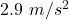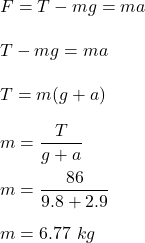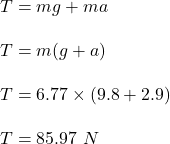## A lamp hangs vertically from a cord in a descending elevator that decelerates at 2.9 m/s2. (a) If the tension in the cord is 86 N, what is t

Question

A lamp hangs vertically from a cord in a descending elevator that decelerates at 2.9 m/s2. (a) If the tension in the cord is 86 N, what is the lamp’s mass? (b) What is the cord’s tension when the elevator ascends with an upward acceleration of 2.9 m/s2?

in progress 0
2 weeks 2021-08-30T18:56:08+00:00 1 Answers 0 views 0

The mass of the lamp is 6.77 kg and tension in the cord is 85.97 N.

Explanation:

Given that,

A lamp hangs vertically from a cord in a descending elevator that decelerates at(a) We need to find the mass of the lamp if the tension in the cord is 86 N.

When the elevator is descending. The net force acting on it is given by :(2) Let T is the cord’s tension when the elevator ascends with an upward acceleration of.

So,So, the mass of the lamp is 6.77 kg and tension in the cord is 85.97 N. Hence, this is the required solution.